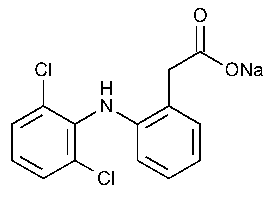# What is the formula for Diclofenac Sodium?

May 28, 2017

The molecular formula for Diclofenac Sodium is ${\text{C"_14"H"_10"Cl"_2"NNaO}}_{2}$.

#### Explanation:

Its structural formula isIts name is sodium {2-[(2,6-dichlorophenyl)amino]phenyl}acetate.

You often see the name written as {2-[(2,6-dichlorophenyl)amino]phenyl}acetic acid, sodium salt

If you mis-spell the name as {2-[(2,6-$\boldsymbol{\text{dicl}}$orophenyl)amino]$\boldsymbol{\text{f}}$enyl}$\boldsymbol{\text{ac}}$etic acid, $\boldsymbol{\text{Na}}$ salt, you can get a glimmer of the trade name.